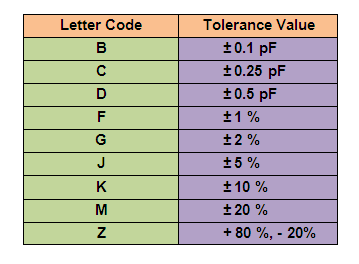Wednesday, March 29, 2023
HomePower ElectronicsCapacitorCapacitor Number Marking - How to Decode with Example

# Capacitor Number Marking – How to Decode with Example

Decoding Capacitor Number Marking to get the capacitance value is a must for electrical circuit designers. It also helps in replacing the capacitor with exact alternate while repairing a PCB. This post will teach you how to decode capacitor number markings with suitable example and that too with simple and easy to remember steps.

In our previous post, we discussed how to decode capacitor marking in color. Was not it interesting? Now let’s learn how to decode capacitor number marking.

## How to Decode Capacitor Number Marking

Mostly, ceramic capacitors come under this category. In normal practice, Ceramic capacitor has a three digit code value on its body. Kindly note that the decoded value of a Ceramic capacitor is always measured in Pico farad (pF).

### Example of a Capacitor

Now, let’s take an example to understand it more easily. Suppose, we have to decode the capacitance value of the below mentioned capacitor.#### Step 1 – First Two Digits of Capacitor Number

The three digit code of the capacitor is 681J. Here, The first 2 digit code gives us the starting capacitance value of the capacitor.Thus, we can easily identify now that in case of capacitor 681J, the first two digit 68 means “the capacitor has starting capacitance value of 68”.

#### Step 2 – Third Digit of Capacitor Number

The third digit says how many zeros we have to add in it to get the actual capacitance value.

Here in the given example, the third digit 1 means “the number of zeroes behind 68 will be one” i.e . the capacitance value will be 690 pF.

#### Step 3 – Fourth letter of Capacitor Number

If there is a letter code after three digits, it denotes the tolerance value of the capacitor. If there is no letter code, then the tolerance value is unknown.

Now , the tolerance as per the letter code is as tabulated below:Thus, we can easily identify now that in case of capacitor 681J, the last letter code J means “the capacitor has a tolerance limit of ± 5 %.

#### Conclusion

Coming to the conclusion, we can now easily decode capacitor marking (number) of most of the capacitors. Here, capacitor 681J means “it has a capacitance value of 680pF ± 5% tolerance.

In some case, we will see only a decimal value written on it. Suppose the decimal value written on the body of a capacitor is 0.01. Then this is the capacitance value in pico Farad. We do not have to convert it.

```Also Read: -
How to Read Capacitor Color Marking Values - Calculation and Identification Codes
Electrolytic Capacitor - Properties, Uses, Capacitance Value and Polarity```Ratna
Ratna is a B.E (Computer Science) and has work experience in UK Mainframe IT industry. She is also an active Web Designer. She is an author, editor and core partner at Electricalfundablog.
RELATED ARTICLES